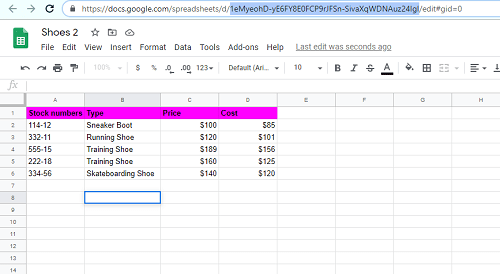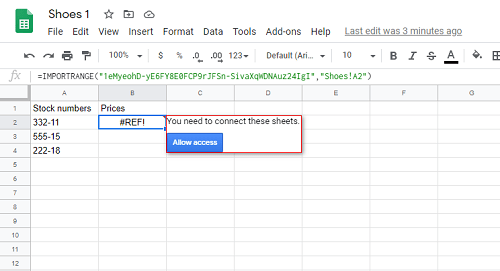# How to Use Vlookup from Another Workbook in Google Sheets

Vlookup is an essential function in spreadsheets, including Google Sheets. It lets you perform vertical lookups by searching for key values in the selected range. This function then returns a value to another column but inside the same row.Vlookup is usually performed between sheets, but you can also use it to pull results for separate workbooks. In this article, we’ll show you how to do it using a practical example.

## Vlookup with Two Workbooks – Step by Step Guide

In this example, we’ll use workbooks containing shoe sale data. As you’re working with two documents, it’s best to set them side by side. Unfortunately, unlike in Microsoft Excel, there’s no side by side view option, so you’ll need to resize windows manually. Another option is to install the Tab Resize app from Chrome Store.1. Copy the URL from the workbook you want to use the data for Vlookup. In our case, that is “Shoes 2”. You only need to copy the part between “d/” and “/edit.”
2. To use the data from the “Shoes 2”, you need to give it access from “Shoes 1”. That is the moment to use IMPORTRANGE function. Use the formula:

In our example, the formula is:

IMPORTRANGE(“1eMyeohD-yE6FY8E0FCP9rJFSn-SivaXqWDNAuz24IgI”,”Shoes!A2″)

Note that if the name your sheet has two or more words, you would have to use a single quote. If the name of the sheet was “Shoe data,” the formula would look like this:

IMPORTRANGE(“1eMyeohD-yE6FY8E0FCP9rJFSn-SivaXqWDNAuz24IgI”,”’Shoes data’!A2″)If you have a lot of data, you may wait for a few minutes before it loads. Now, click on “Allow access” to connect these sheets from different workbooks.Now that you’ve been connected, you can use Vlookup. In B2 field on “Shoes 1”, delete the formula we just implemented, and type the following:

VLOOKUP(A2,IMPORTRANGE(“1eMyeohD-yE6FY8E0FCP9rJFSn-SivaXqWDNAuz24IgI”,”Shoes!A2:D6″),3,0)

We used the IMPORTRANGE function with the spreadsheet key and sheet name from “Shoes.” Then we provided the range, index, and the “0” at the end as this data isn’t sorted.

The formula is:

VLOOKUP(search_key, IMPORTRANGE (spreadsheet_key, range string), index, is_sorted

Note that the range you provided “, “Shoes!A2:D6” is a string, unlike all the other cell references. In this case, we don’t need to lock the range because it’s text, and it won’t change.

Also, we defined range as “A2:D6”, but if you add the data into D7, you’ll need to change it to “A2:D7”. However, it’s better to input “A2:D”. This way, you’re not specifying the row that Google Sheets will check them all. The advantage of this is that if we add more items, we don’t need to update the formula

## Vlookup in Google Sheets – How to Avoid Errors

If you’ve used Vlookup in Microsoft Excel, you might get confused, as this function works a bit differently on Google Sheets. Here’s what you need to know before we start.

Vlookup in Google Sheet isn’t case sensitive by default, so it doesn’t make a difference between lowercase and uppercase. If you need case-sensitive Vlookup, use this formula:

ArrayFormula(INDEX(return_range, MATCH (TRUE,EXACT(lookup_range, search_key),0)))

If the is_sorted argument is set to TRUE or isn’t present, you’ll need to use ascending order on the first column. By doing so, Vlookup will perform a faster search that only works when the data is sorted.

If you want a partial match, you can use two wildcard characters: an asterisk (*) and the question mark (?).

By default, Vlookup in Google Sheets always searches the leftmost column. To override it, use the formula

INDEX (return_range, MATCH(search_key, lookup_range, 0))

## The Syntaxes to Use

To better understand this guide, here’s a list of syntaxes we’ve used:

Search_key – This is the value we search for, also called a unique identifier.

Range – Select two or more columns to perform the search.

Index – This is the number of the column that has the value you need to import into another spreadsheet.

Is_sorted – there are only two values here, and FALSE is the default.

Use TRUE if the columns need to be sorted from smallest to largest or A to Z. This way, the Vlookup formula might not return the exact match. Instead, you’ll get the approximate result that is less than search_key. If there are none, you will receive the error message.

Use FALSE if you don’t require sorting. Vlookup will look only for exact matches and return an error if it doesn’t find any. If there are two or more equal values, Vlookup will use the first.## Successfully Connecting the Workbooks

As you have hopefully learned, connecting different workbooks in Google Sheets with Vlookup is a straightforward process when using the IMPORTRANGE function. While the VLookup function works the same way in Excel, these apps won’t work together. Because of its intuitive formulas and features not present in Microsoft’s spreadsheet alternative, Google Sheets might be the best solution for working with multiple workbooks and sheets.

How often do you use Vlookup function in Google Sheets? Do you often work on two or more workbooks at the same time? Let us know in the comments section below.

Disclaimer: Some pages on this site may include an affiliate link. This does not effect our editorial in any way.

Todays Highlights